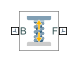# Spring and Damper Force

Force proportional to the distance and relative velocity between two frame origins

## Library

Forces and Torques

•## Description

This block represents a linear spring and damper force pair acting reciprocally between base and follower frame origins. The two forces in the pair have equal magnitude but opposite directions. One force acts on the base frame origin, along the vector connecting follower to base frame origins. The other force acts on the follower frame origin, along the vector connecting base to follower frame origins.

The magnitude of the spring force component is proportional to the distance between base and follower frame origins. This distance is the length of the straight line segment connecting the two origins. The magnitude of the damper force component is proportional to the relative velocity of the follower frame origin with respect to the base frame.

## Parameters

Natural Length

Enter the equilibrium distance between the base and follower frame origins. This is the distance at which the magnitude of the spring force is zero. The default value is `0`. Select or enter a physical unit.

Spring Stiffness

Enter the value of the linear spring constant. The value must be greater than or equal to zero. The default value is zero. Select or enter a physical unit.

Damping Coefficient

Enter the value of the linear damping coefficient. The value must be greater than or equal to zero. The default value is zero. Select or enter a physical unit.

Sense Force

Select to sense the signed magnitude of the spring and damper force acting between the two frame origins. The block exposes an additional physical signal port to output the force signal. The output signal is a scalar value. This value is positive if the force is repulsive; it is negative if the force is attractive.

## Ports

The block contains frame ports B and F, representing base and follower frames, respectively.

Selecting the Sense Force check box in the block dialog box adds a physical signal port, fm.

## Version History

Introduced in R2012a